# Exponentiation + multiplication principle - math problems

#### Number of problems found: 8

• Cube constructionA 2×2×2 cube is to be constructed using 4 white and 4 black unit cube. How many different cubes can be constructed in this way? ( Two cubes are not different if one can be obtained by rotating the other. )
• Boys and girlsThere are eight boys and nine girls in the class. There were six children on the trip from this class. What is the probability that left a) only boys b) just two boys
• Area codes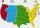How many 6 digit area codes are possible if the first number can't be zero?
• No. of divisorsHow many different divisors have number 13 4 * 2 4?
• Events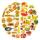Event P has probability of 0.84. What is the probability that the event P occurs in 3, 5, 7 try.
• Subsets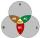How many are all subsets of set C = (97, 67, 66, 18, 59, 64)?
• Bits, bytes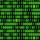Calculate how many different numbers can be encoded in 16-bit binary word?
• Morse alphabet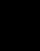Calculate how many words of Morse code to create compiling dashes and dots in the words of one to four characters.

We apologize, but in this category are not a lot of examples.
Do you have an exciting math question or word problem that you can't solve? Ask a question or post a math problem, and we can try to solve it.

We will send a solution to your e-mail address. Solved examples are also published here. Please enter the e-mail correctly and check whether you don't have a full mailbox.

Exponentiation - math problems. Multiplication principle - math problems.